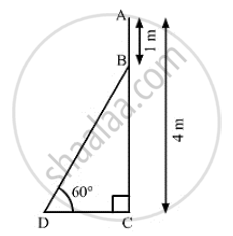# An Electrician Has to Repair an Electric Fault on a Pole of Height 4 Meters. He Needs to Reach a Point 1 Meter Below the Top of the Pole to Undertake the Repair Work. - Mathematics

An electrician has to repair an electric fault on a pole of height 4 meters. He needs to reach a point 1 meter below the top of the pole to undertake the repair work. What should be the length of the ladder that he should use, which when inclined at an angle of 60° to the horizontal would enable him to reach the required position?

#### SolutionLet AC be the pole and BD be the ladder

We have,
AC = 4m, AB = 1m and ∠BDC = 60°
And, BC = AC - AB = 4-1= 3m
In ΔBDC,

sin 60° = (BC)/(BD)

⇒sqrt(3)/2 =3/(BD)

⇒ BD = (3xx2)/sqrt(3)

⇒ BD = 2 sqrt(3)

⇒ BD = 2 xx 1.73

∴ BD = 3.46 m

So, he should use 3.46 m long ladder to reach the required position.

Concept: Heights and Distances
Is there an error in this question or solution?
Chapter 14: Height and Distance - Exercises

#### APPEARS IN

RS Aggarwal Secondary School Class 10 Maths
Chapter 14 Height and Distance
Exercises | Q 32

Share